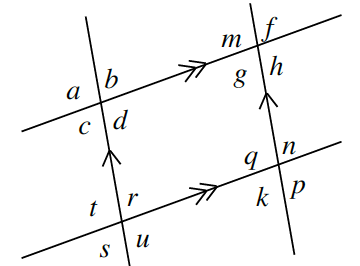### Home > GC > Chapter 6 > Lesson 6.1.3 > Problem6-29

6-29.

Multiple Choice: Given the diagram at right, which of the statements below is not necessarily true?

1. $a = d$

1. $d + r = 180°$

1. $u = n$

1. $t = m$

1. These all must be true.Analyze each statement. Which one(s) are true?
Which one(s) are false?

(c). $∠u$ and $∠n$ will be supplementary, so this is false.